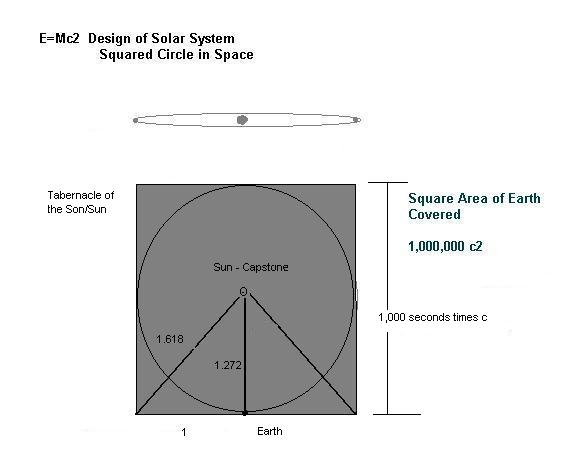When it comes to the divine design of our Solar System,  we know or found out in â€¦one billionth of a second or a nano
second, light travels one foot.

Therefore in 1 second, it travels a billion feet
In a thousand seconds the time to travel across the
Tabernacle of the Sun/Son it travels a trillion feet.
Times that by 1000 more seconds to calculate the square area of the Tabernacle of the Sun and you get 1000 trillion

http://gracethrufaith.com/ikvot-hamashiach/the-great-pyramid-of-giza/

The Sacred Cubit was the unit of measure used in building the Great Pyramid. Unlike the standard and royal cubits referenced
in the Bible, its length is 25 pyramid inches, which are about the same as the inch used today in the US. These units of measure
were carved into the wall, and can serve to decode the pyramidâ€™s dimensions. For instance, multiplying the sacred cubit
times 10 million equals the polar radius of earth. Multiplying the total weight of the Pyramid by 1000 trillion equals the weight
of planet Earth.

Hmmm...... thereâ€™s our number 1000 trillion x Giza weight equals the weight of the EARTHâ€¦ thatâ€™s definitely an
interesting MASS and the mass we were looking for as it is the orbiting planet we wanted the mass for. And the center of the
Pyramid or Tabernacle of the Son/Sun is the capstone.

So lets see if that fits the dimensions we are used to. Meaning the distances for the diameter of the Sun and its distance from
the Earth> if they match, then we have convergence and the probable solution.

The distance from our Son to the earth, its Bride is 93,000,000

The diameter of the Sun is approximately 880,000 miles although its gaseous and this is only an approximation of its outer
gases.

880,000/93,000,000 = approximately .01 or 1 percent approximately, so lets see if this approximates a â€˜phi pyramidâ€™ or
Giza demensions between base and capstone.

http://www.davidjayjordan.com/Capstoneand1-56.html

But what was the actual size of the golden capstone that was placed atop the Great Pyramid, the initiation chamber to the
stars. Well according to the Great Pyramid Facts and Statistics Web- http://www.crystalinks.com/gpstats.html The capstone
was 103 inches in height , approximately 8.58 feet in height with a base side of 13.5 feet, But this fits nicely into the Holy of
Holies which was 15 feet by 15 feet by 15 feet. In other words the Capstone would fit perfectly into the Holy of Holies, for
remember the ARK and the King's Sacrophagus or initiation coffin were exactly the same size. SEE Giza Saccophagus.

But the Kings chamber is not at the height of the phi pyramid nor is the ARK of the Covenant at the top of the Holy of Holiesâ
€¦. But lower. The standing man inside the Holy of Holies would have a heart at about 5 feet from the base.

Hence the ratio of 5â€™/500â€™ = .01 or one percent and again would be in the range of the Solar System dimensions and a
confirmation of distances. And who is the capstone, the stone the builders rejected â€¦ JESUS

Jesus is the capstone of the Solar System Tabernacle of the Sun/Son Pyramid. His heart is in the center and the Earth swings
around it in a harmonic circle as our calendar for the year (SEE Pythagorus Comma) So that also meshes with symbolic truths.

And besides as a swinging pendulum or mass on a string equals mass times velocity  = mv equals momentum, where Earth
equals the MASS and velocity equals the speed of light THEN if we also multiply again because the light passes to all the area
ior square or cube of the Tabernacle, Then the equation can look like mvv or mv2

So that the momentum or energy of the Tabernacle appears to be Momentum Energy in all the Tabernacle =mc2  or  E=mc2
E=mc2   Design of Solar System